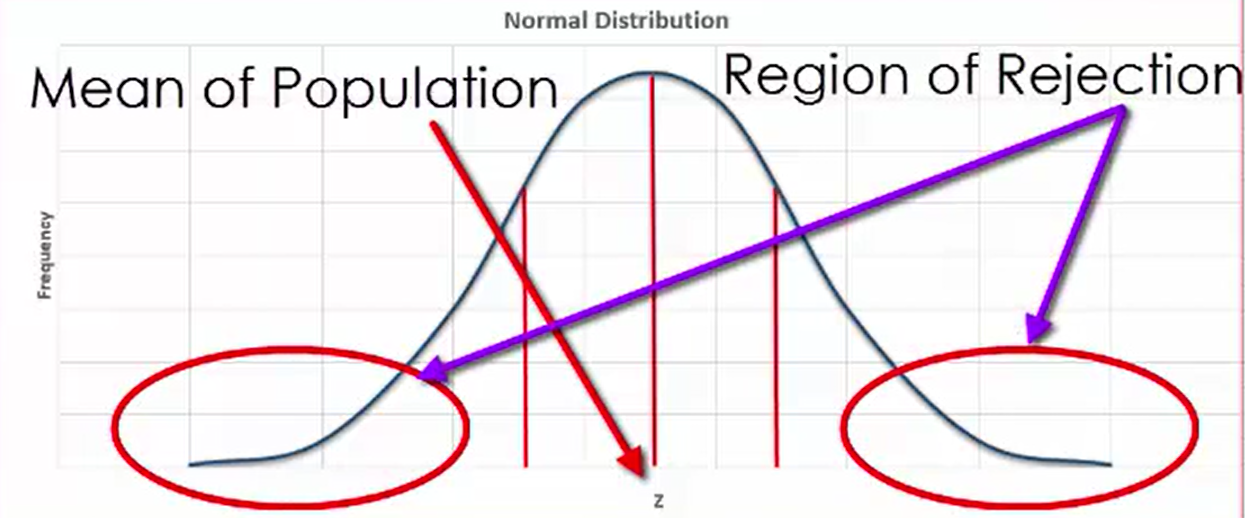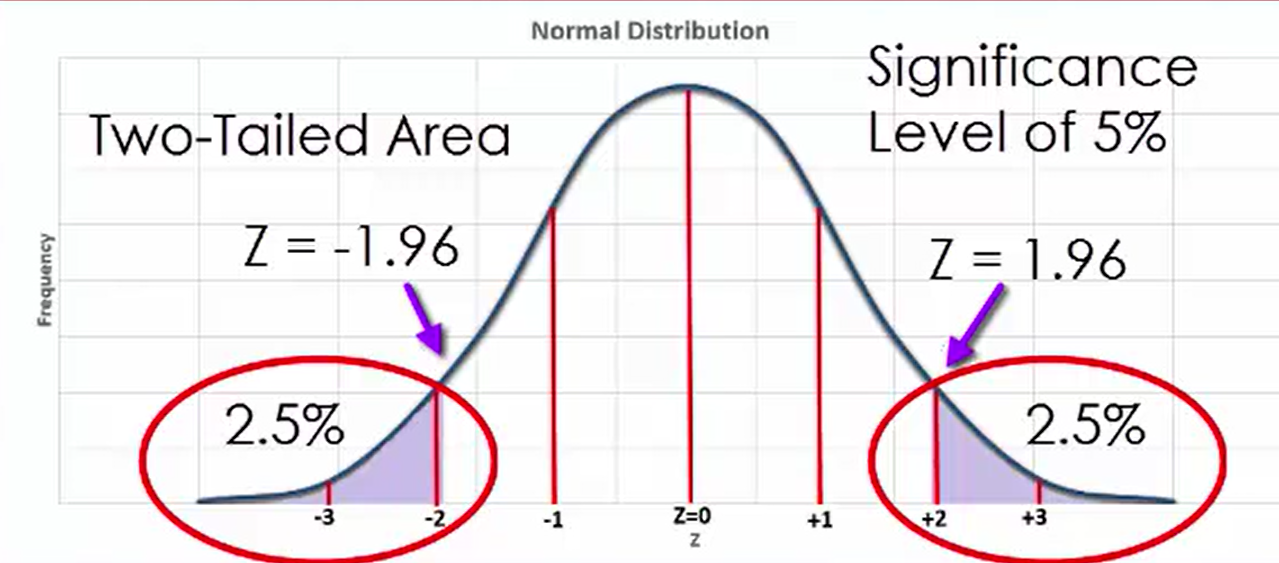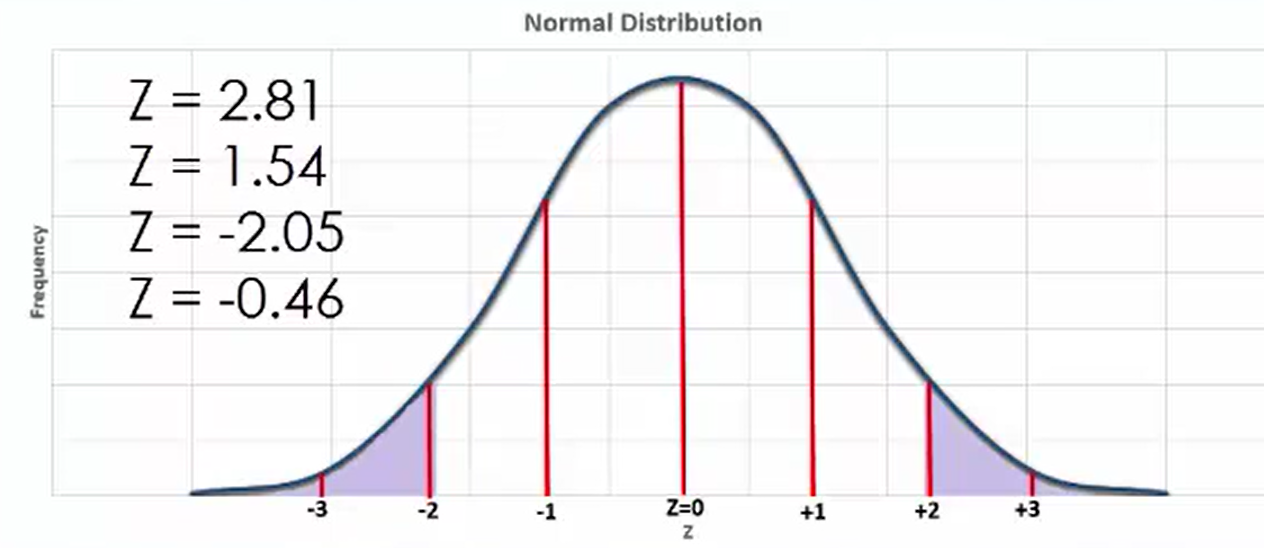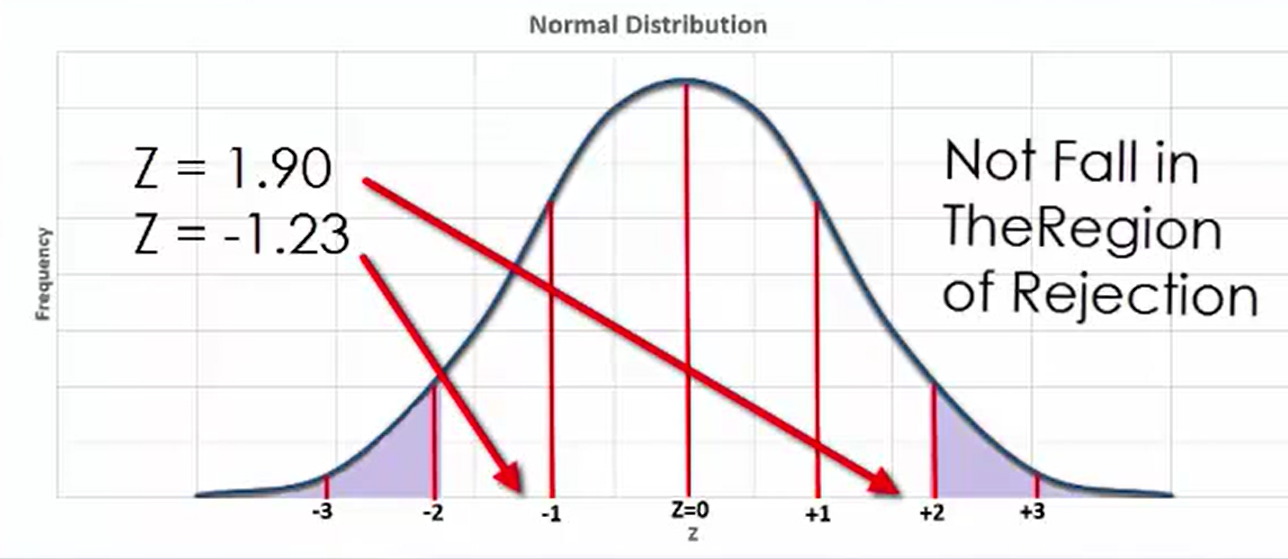+
Introduction to Significance Levels
Interpret Inferential Statistics
0
of 0 possible points

# Introduction to Significance Levels

Author: Sophia Tutorial
##### Description:

Determine if a given z-score lies in the region of rejection.

(more)

Sophia’s self-paced online courses are a great way to save time and money as you earn credits eligible for transfer to many different colleges and universities.*

No credit card required

37 Sophia partners guarantee credit transfer.

299 Institutions have accepted or given pre-approval for credit transfer.

* The American Council on Education's College Credit Recommendation Service (ACE Credit®) has evaluated and recommended college credit for 32 of Sophia’s online courses. Many different colleges and universities consider ACE CREDIT recommendations in determining the applicability to their course and degree programs.

Tutorial
what's covered
This lesson discusses significance levels. By the end of this lesson, you should be able to identify the significance level in a graph of a sampling distribution. This lesson covers:
1. Sampling and Significance Levels
2. Determining a Region of Rejection

## 1. Sampling and Significance Levels

The benefit of taking random samples from a population is that it enables us to get a more representative estimation of what the population actually looks like. When you draw a lot of random samples, the result is having a sample mean for each sample, or a lot of sample means. In the event that the sample sizes are large enough, the sample means will be normally distributed and centered on the mean of the population.

When sampling data, it is helpful to know which sampling means are very large and which are very small. This could be done by selecting a region on a normal distribution that falls far away from the mean in both directions. This area on the distribution is referred to as the region of rejection. It represents that part of the distribution in which the results are not likely to be due to chance.

This area of the region is known as the significance level, a level that is typically chosen to be 5%. Sample means that fall into this region of rejection are generally considered vastly different from the population mean and are unlikely to occur as sample means.## 2. Determining a Region of Rejection

The areas of the region of rejection lie in the areas to the left of z = -1.96 and to the right of z = 1.96. This is what is called a two-tailed area. One tail lies to the far right of the distribution and the other to the far left, with each comprising 2.5% of the total area under the curve. When considering a z-table, these 2.5% areas translate to z-scores of less than - 1.96 and greater than 1.96. This 5% combined area of the region of rejection relates to the significance level of 5%.try it
Suppose you have an example of a normally distributed variable in which there are four observation that interest you. If these four values translate to the z-scores you see below, which ones lie in the region of rejection?The z-score of 2.81 falls to the right of z = 1.96 and the z-score of -2.05 falls to the left of z = -1.96, so both scores are in the region of rejection. However, the z-scores of 1.54, which does not fall to the right of z = 1.96, and -0.46, which does not fall to the left of z= -1.96, do not fall in the region of rejection.

When a z-value lies in the region of rejection, it is understood that the corresponding sample mean varies significantly from the population mean. The z-scores of 1.90 and -1.23 would not fall in the region of rejection, which means that the sample means corresponding to these z-scores would not be rejected, because they do not vary significantly from the population mean.summary

Source: This work is adapted from Sophia author Dan Laub.

Rating